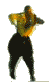# Integer sequence review: A005114

The Online Encyclopedia of Integer Sequences contains over 200,000 sequences. It contains classics, curios, thousands of derivatives entered purely for completeness’s sake, short sequences whose completion would be a huge mathematical achievement, and some entries which are just downright silly.

For a lark, David and I have decided to review some of the Encyclopedia’s sequences. We’re rating sequences on four axes: NoveltyAestheticsExplicability and Completeness.

Following last week’s palaver, we’re going to do our best to be serious this time. Game faces on. David promises there will actually be some maths in this sequence.

#### A005114 Untouchable numbers: impossible values for sum of aliquot parts of $n$.

2, 5, 52, 88, 96, 120, 124, 146, 162, 188, 206, 210, 216, 238, 246, 248, 262, 268, 276, 288, 290, 292, 304, 306, 322, 324, 326, 336, 342, 372, 406, 408, 426, 430, 448, 472, 474, 498, 516, 518, 520, 530, 540, 552, 556, 562, 576, 584, 612, 624, 626, 628, 658, ...

Christian: Explain?

David: A number, $k$, is untouchable if there exists no $n$ such that the sum of $n$’s proper divisors (its divisors, including $1$ but excluding itself) is equal to $k$. In other words, $k \neq \sigma(n) – n$.

Christian: Klaro. So we might as well give it its explicability score now.

### Explicability

Christian: Five!

David: Not everyone (statisticians) will understand what a proper divisor is. We need to take a point off.

$\frac{4}{5}$

### Novelty

Christian: It’s new to me, but old to the OEIS – it was in the original paper version as M1552.

David: Google told me it’s been known “since antiquity”. And you told me that’s since the ancient Romans. The five is pretty novel. Did you know that it’s conjectured that 5 is the only odd untouchable number?

Christian: That isn’t novel but it is interesting. Please do tell me more.

David: If the superduperstrong Goldbach conjecture holds…

Christian: ?

David: Every even integer greater than 6 is the sum of two distinct primes.

Christian: Carry on.

David: … then five is the only odd untouchable number. Suppose that $k = 2n+1$ is an odd untouchable number. Then $2n$ is an even number, and if it’s bigger than 6, then $2n$ is the sum of two distinct primes $p$ and $q$, i.e. $2n = p+q$. And $k = 2n+1 = p+q+1$.

Then the proper divisors of $pq$ are $1, p$ and $q$. So $k$ is the sum of the proper divisors of $pq$, so it isn’t untouchable.

Christian: CONTRADICTIO IN EXCELSIS ET TUTAMEN ET CETERA

David: Christian?

Christian: What?

David: Can you prove that five is untouchable?

(time passes)

Christian: I thought I could but I can’t. Anyway, we need a Novelty score. I say it’s really old.

David: Three.

Christian: It’s really old though.

David: So is the number 7 and we gave that five.

Christian: But these are more serious times. We need to weigh this up properly.

David: We’ve only recently discovered the 53rd term.

Christian: Fine. Meet you halfway?

David: YOU DIDN’T KNOW WHAT THEY WERE BEFORE WE STARTED THIS.

Christian: I don’t know plenty of old things. Like your ma.

David: That’s it, I’m leaving.$\frac{1}{5}$

### Aesthetics

Shrugs all round (David was shrugging from a polite distance)

Christian: Middle, I suppose. Nothing for or against it.$\frac{3}{5}$

### Completeness

Christian: There are infinitely many, thanks to our old pal Erdős, but we only know 53 of them for definite. That’s pretty much entirely incomplete. I bet if David was here, he’d quantify the completeness by considering the limit of $\frac{53}{n}$ as $n$ tends to infinity.$\frac{0}{5}$

### Total score

$\frac{4+1+3+0}{20} = \frac{8}{20} = \frac{2}{5}$

Christian: We’ve made a huge mistake. This is a very good sequence and we’ve given it a rubbish score.

David: MC Hammer is 51. Next year, his age will be an untouchable number.

### SCORE AMENDED DUE TO NEW EVIDENCE!

$\frac{51}{52}$## About the authors

•#### Christian Lawson-Perfect

Mathematician, koala fan, Aperiodical editor. Usually found paddling in the North Sea, or fiddling with computers.
•#### David Cushing

Mathematician / magician / origami enthusiast. Wanted for fraud in at least one branch of Subway.

### One Response to “Integer sequence review: A005114”

1.Robert Anderson

Deserves an extra point for using the word aliquot.